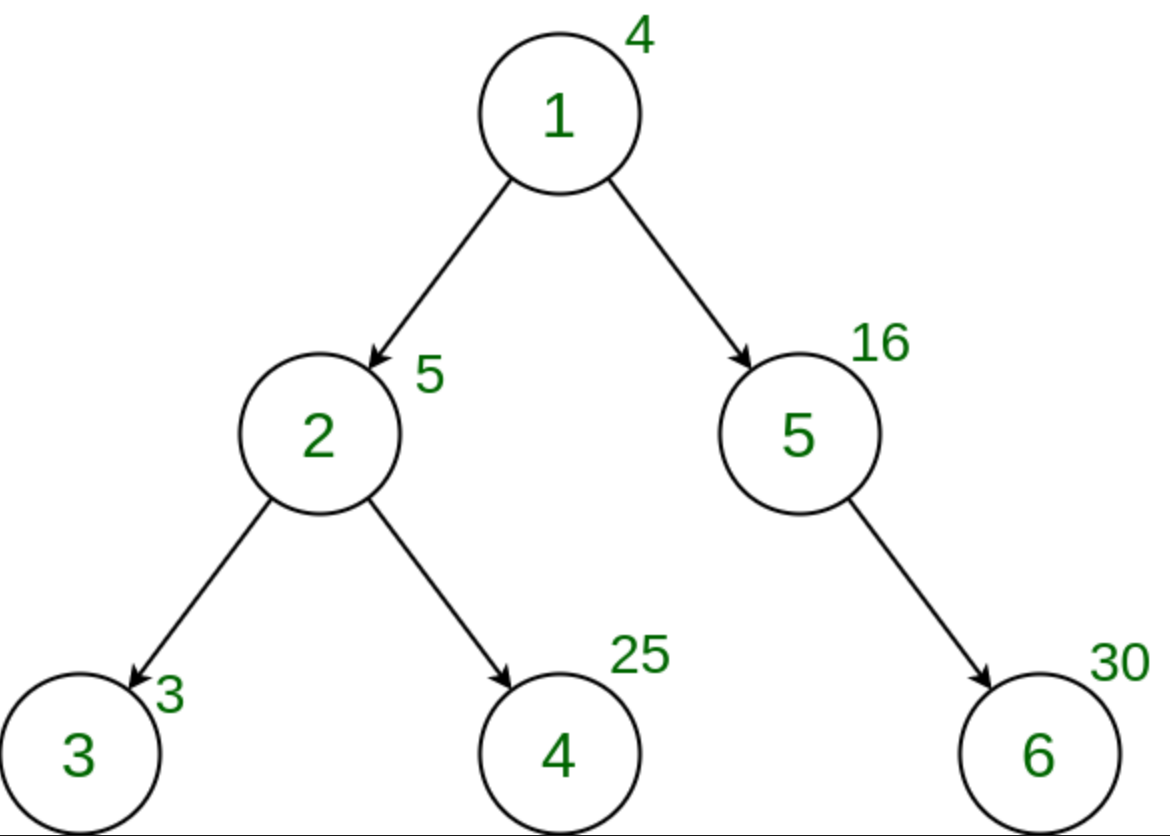Related Articles

# Count the nodes in the given Tree whose weight is a Perfect Number

• Last Updated : 03 Jun, 2021

Given a tree, and the weights of all the nodes, the task is to count the number of nodes whose weight is a Perfect number.

A perfect number is a positive integer that is equal to the sum of its proper divisors

Examples:

Input:Output:
Explanation:
There is no node with a weight that is a perfect number.

Approach:
In order to solve this problem, we perform Depth First Search(DFS) Traversal on the tree and for every node, check if its weight is a Perfect Number or not. We keep on incrementing the counter every time such a weight is obtained. The final value of that counter after the completion of the entire tree traversal is the answer.
Below is the implementation of the above approach:

## C++

 `// C++ implementation to Count the nodes in the``// given tree whose weight is a Perfect Number` `#include ``using` `namespace` `std;` `int` `ans = 0;``vector<``int``> graph;``vector<``int``> weight(100);` `// Function that returns true if n is perfect``bool` `isPerfect(``long` `long` `int` `n)``{``    ``// Variable to store sum of divisors``    ``long` `long` `int` `sum = 1;` `    ``// Find all divisors and add them``    ``for` `(``long` `long` `int` `i = 2; i * i <= n; i++) {``        ``if` `(n % i == 0) {``            ``if` `(i * i != n)``                ``sum = sum + i + n / i;``            ``else``                ``sum = sum + i;``        ``}``    ``}` `    ``// Check if sum of divisors is equal to``    ``// n, then n is a perfect number``    ``if` `(sum == n && n != 1)``        ``return` `true``;` `    ``return` `false``;``}` `// Function to perform dfs``void` `dfs(``int` `node, ``int` `parent)``{` `    ``// If weight of the current node``    ``// is a perfect number``    ``if` `(isPerfect(weight[node]))``        ``ans += 1;` `    ``for` `(``int` `to : graph[node]) {``        ``if` `(to == parent)``            ``continue``;``        ``dfs(to, node);``    ``}``}` `// Driver code``int` `main()``{` `    ``// Weights of the node``    ``weight = 5;``    ``weight = 10;``    ``weight = 11;``    ``weight = 8;``    ``weight = 6;` `    ``// Edges of the tree``    ``graph.push_back(2);``    ``graph.push_back(3);``    ``graph.push_back(4);``    ``graph.push_back(5);` `    ``dfs(1, 1);``    ``cout << ans;` `    ``return` `0;``}`

## Java

 `// Java implementation to Count the nodes in the``// given tree whose weight is a Perfect Number` `import` `java.util.*;` `class` `GFG{` `static` `int` `ans = ``0``;``static` `Vector []graph = ``new` `Vector[``100``];``static` `int` `[]weight = ``new` `int``[``100``];` `// Function that returns true if n is perfect``static` `boolean` `isPerfect(``int` `n)``{``    ``// Variable to store sum of divisors``    ``int` `sum = ``1``;` `    ``// Find all divisors and add them``    ``for` `(``int` `i = ``2``; i * i <= n; i++) {``        ``if` `(n % i == ``0``) {``            ``if` `(i * i != n)``                ``sum = sum + i + n / i;``            ``else``                ``sum = sum + i;``        ``}``    ``}` `    ``// Check if sum of divisors is equal to``    ``// n, then n is a perfect number``    ``if` `(sum == n && n != ``1``)``        ``return` `true``;` `    ``return` `false``;``}` `// Function to perform dfs``static` `void` `dfs(``int` `node, ``int` `parent)``{` `    ``// If weight of the current node``    ``// is a perfect number``    ``if` `(isPerfect(weight[node]))``        ``ans += ``1``;` `    ``for` `(``int` `to : graph[node]) {``        ``if` `(to == parent)``            ``continue``;``        ``dfs(to, node);``    ``}``}` `// Driver code``public` `static` `void` `main(String[] args)``{` `    ``for` `(``int` `i = ``0``; i < graph.length; i++)``        ``graph[i] = ``new` `Vector();``        ` `    ``// Weights of the node``    ``weight[``1``] = ``5``;``    ``weight[``2``] = ``10``;``    ``weight[``3``] = ``11``;``    ``weight[``4``] = ``8``;``    ``weight[``5``] = ``6``;` `    ``// Edges of the tree``    ``graph[``1``].add(``2``);``    ``graph[``2``].add(``3``);``    ``graph[``2``].add(``4``);``    ``graph[``1``].add(``5``);` `    ``dfs(``1``, ``1``);``    ``System.out.print(ans);` `}``}` `// This code contributed by Princi Singh`

## Python3

 `# Python3 implementation to``# Count the Nodes in the given``# tree whose weight is a Perfect``# Number` `graph ``=` `[[] ``for` `i ``in` `range``(``100``)]``weight ``=` `[``0``] ``*` `100``ans ``=` `0` `# Function that returns``# True if n is perfect``def` `isPerfect(n):``  ` `    ``# Variable to store``    ``# sum of divisors``    ``sum` `=` `1``;` `    ``# Find all divisors``    ``# and add them``    ``i ``=` `2``;``    ` `    ``while``(i ``*` `i < n):``        ``if` `(n ``%` `i ``=``=` `0``):``            ``if` `(i ``*` `i !``=` `n):``                ``sum` `=` `sum` `+` `i ``+` `n ``/` `i;``            ``else``:``                ``sum` `=` `sum` `+` `i;``        ``i ``+``=` `1``;` `    ``# Check if sum of divisors``    ``# is equal to n, then n is``    ``# a perfect number``    ``if` `(``sum` `=``=` `n ``and` `n !``=` `1``):``        ``return` `True``;` `    ``return` `False``;` `# Function to perform dfs``def` `dfs(Node, parent):``  ` `    ``# If weight of the current``    ``# Node is a perfect number``    ``global` `ans;``    ` `    ``if` `(isPerfect(weight[Node])):``        ``ans ``+``=` `1``;` `    ``for` `to ``in` `graph[Node]:``        ``if` `(to ``=``=` `parent):``            ``continue``;``        ``dfs(to, Node);` `# Driver code``# Weights of the Node``weight[``1``] ``=` `5``;``weight[``2``] ``=` `10``;``weight[``3``] ``=` `11``;``weight[``4``] ``=` `8``;``weight[``5``] ``=` `6``;` `# Edges of the tree``graph[``1``].append(``2``);``graph[``2``].append(``3``);``graph[``2``].append(``4``);``graph[``1``].append(``5``);` `dfs(``1``, ``1``);``print``(ans);` `# This code is contributed by 29AjayKumar`

## C#

 `// C# implementation to count the``// nodes in the given tree whose``// weight is a Perfect Number``using` `System;``using` `System.Collections.Generic;` `class` `GFG{` `static` `int` `ans = 0;``static` `List<``int``> []graph = ``new` `List<``int``>;``static` `int` `[]weight = ``new` `int``;` `// Function that returns true``// if n is perfect``static` `bool` `isPerfect(``int` `n)``{``    ` `    ``// Variable to store sum of``    ``// divisors``    ``int` `sum = 1;` `    ``// Find all divisors and add them``    ``for``(``int` `i = 2; i * i <= n; i++)``    ``{``       ``if` `(n % i == 0)``       ``{``           ``if` `(i * i != n)``               ``sum = sum + i + n / i;``           ``else``               ``sum = sum + i;``       ``}``    ``}` `    ``// Check if sum of divisors is equal``    ``// to n, then n is a perfect number``    ``if` `(sum == n && n != 1)``        ``return` `true``;``    ``return` `false``;``}` `// Function to perform dfs``static` `void` `dfs(``int` `node, ``int` `parent)``{` `    ``// If weight of the current node``    ``// is a perfect number``    ``if` `(isPerfect(weight[node]))``        ``ans += 1;` `    ``foreach``(``int` `to ``in` `graph[node])``    ``{``        ``if` `(to == parent)``            ``continue``;``        ``dfs(to, node);``    ``}``}` `// Driver code``public` `static` `void` `Main(String[] args)``{` `    ``for``(``int` `i = 0; i < graph.Length; i++)``       ``graph[i] = ``new` `List<``int``>();``        ` `    ``// Weights of the node``    ``weight = 5;``    ``weight = 10;``    ``weight = 11;``    ``weight = 8;``    ``weight = 6;` `    ``// Edges of the tree``    ``graph.Add(2);``    ``graph.Add(3);``    ``graph.Add(4);``    ``graph.Add(5);` `    ``dfs(1, 1);``    ``Console.Write(ans);``}``}` `// This code is contributed by amal kumar choubey`

## Javascript

 ``
Output:
`1`

Complexity Analysis:

Time Complexity: O(N*logV), where V is the maximum weight of a node in the tree

In DFS, every node of the tree is processed once, and hence the complexity due to the dfs is O(N) if there are total N nodes in the tree. Also, while processing every node, in order to check if the node value is a perfect number or not, the isPerfect(V) function where V is the weight of the node is being called and this function has a complexity of O(logV), hence for every node, there is an added complexity of O(logV). Therefore, the time complexity is O(N*logV).

Auxiliary Space: O(1).

Any extra space is not required, so the space complexity is constant.My Personal Notes arrow_drop_up